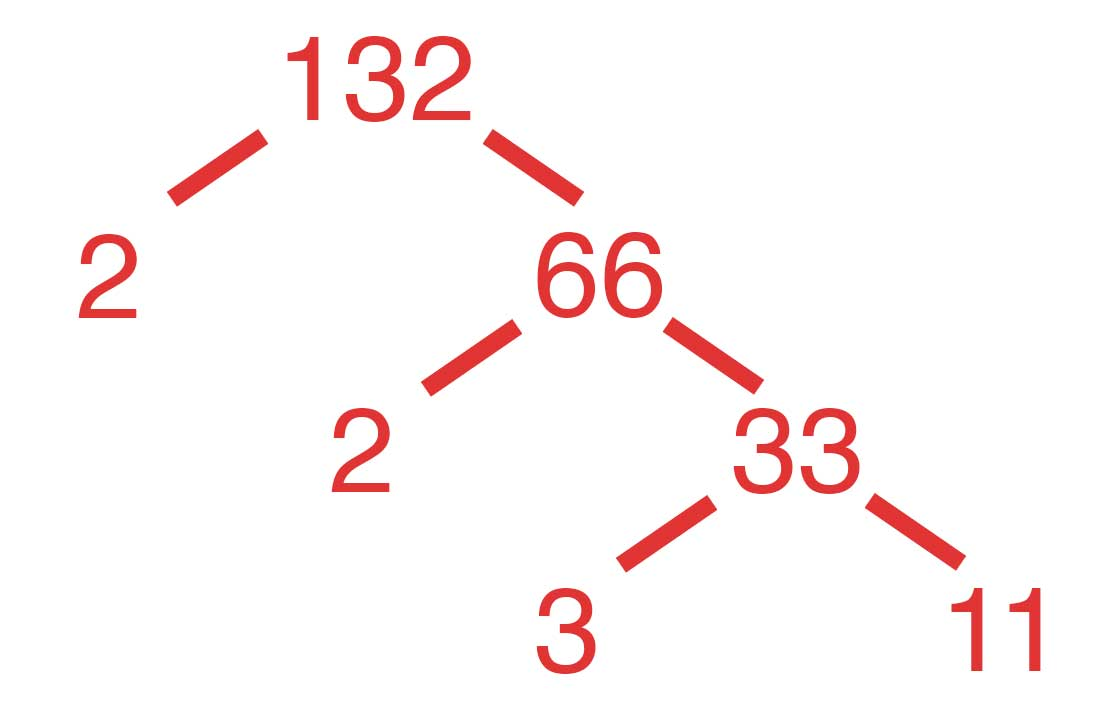# How to find prime factors of a number in Java

### Finding Prime Factors of a Number

A prime number is a counting number greater than 1 that can only be divided by 1 and the number itself.

For example, 2,3,5,7,11,13,… are prime numbers.

Prime factors the multiset of prime numbers whose product is the number.

This articles discusses Java programs to find prime factors of a given number.

For example,

If input number is 12, then output should be “2 2 3”.
If input number is 132, then output should be “2 2 3 11”.### Java programs to find prime factors

```package com.topjavatutorial;

import java.util.ArrayList;
import java.util.List;
import java.util.Scanner;

public class PrimeFactors {

public static void main(String[] args) {
Scanner sc = new Scanner(System.in);
System.out.println("Enter a number");
int num = sc.nextInt();
sc.close();
List<Integer> factors = primeFactors(num);
System.out.println("The Prime Factors of " + num + " are : " + factors);

}

private static List<Integer> primeFactors(int n) {
List<Integer> factors = new ArrayList<Integer>();
for (int factor = 2; factor * factor <= n; factor++) {

// if i is a factor, add it to the list and divide number by i
while (n % factor == 0) {
System.out.print(factor + " ");
n = n / factor;
}
}
// If there's anything left of the number, it is a factor too
if (n > 1) {
System.out.println(n);
} else
System.out.println();
return factors;
}
}

```

#### Output :

``````
Enter a number
90
2 3 3 5
The Prime Factors of 90 are : [2, 3, 3, 5]
``````

Although the above algorithm is simple, its not efficient.

It loops upto the number to find prime factors. However, we just need to loop of to sqrt(number).

Also, every time we find a factor, we can divide the number by it to modify the upper bound until which we need to check.

Again, if a number is divisible by 2, it will be divisible by any even number. So, we just need to check divisibility by 2 and odd numbers.

Here is a modified version of the algorithm :

```  private static List<Integer> primeFactors2(int num) {
List<Integer> factors = new ArrayList<Integer>();
int i = 2;
while (num % i == 0) {
num = num / 2;
}
i = 3;
while (i <= Math.sqrt(num)) {
while (num % i == 0) {
System.out.println("Add " + i + " as factor");
num = num / i;
}
i = i + 2;
}
// Anything left as a factor
if (num > 1) {
System.out.println("Add " + num + " as factor");
}
return factors;
}
```